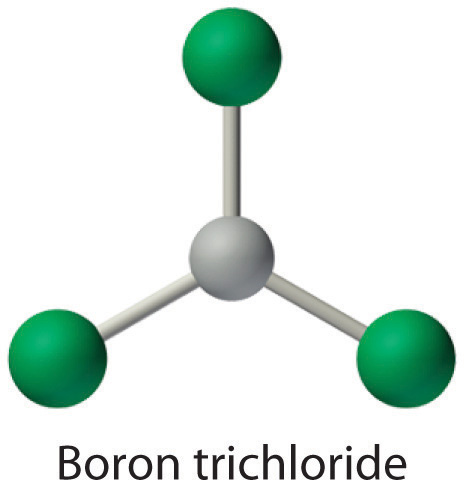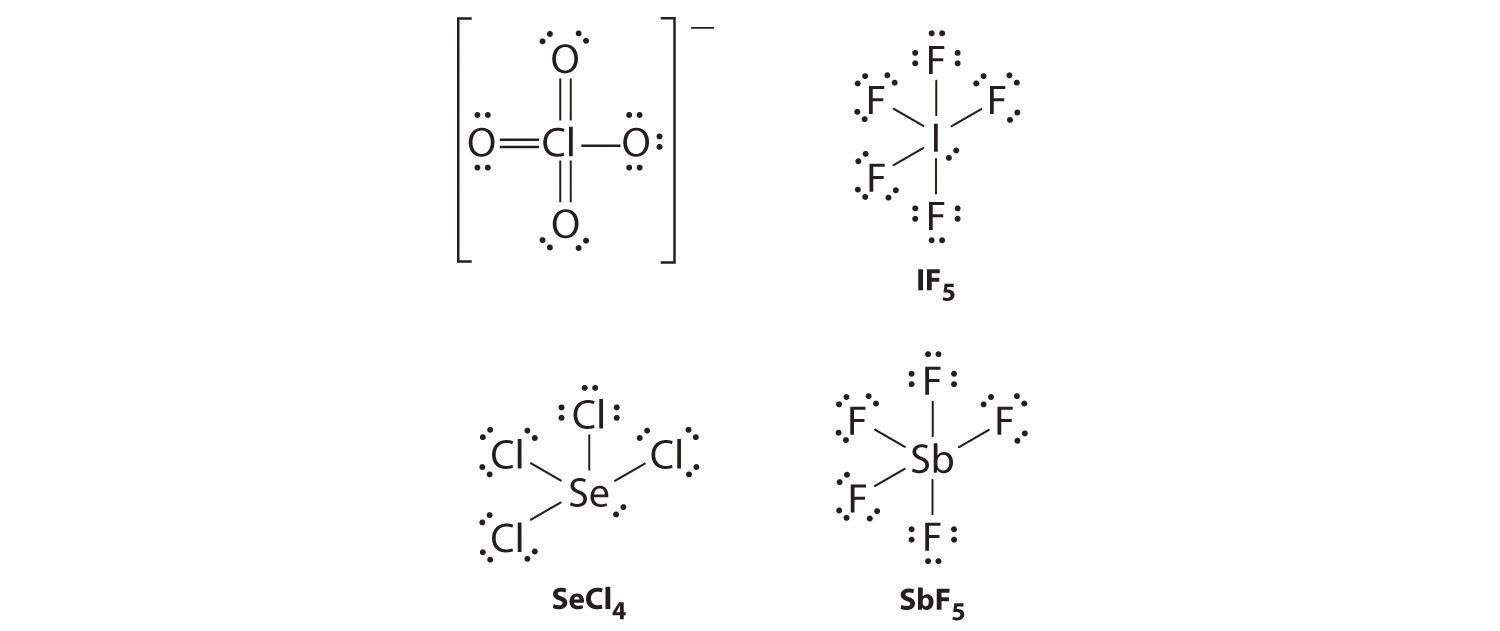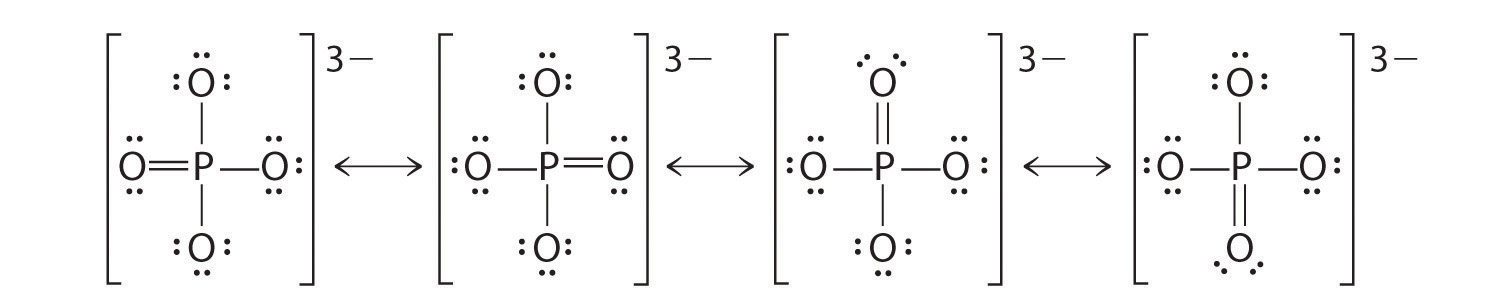## 8.6 Exceptions to the Octet Rule

### Learning Objective

1. To assign a Lewis dot symbol to elements not having an octet of electrons in their compounds.

Lewis dot structures provide a simple model for rationalizing the bonding in most known compounds. However, there are three general exceptions to the octet rule: (1) molecules, such as NO, with an odd number of electrons; (2) molecules in which one or more atoms possess more than eight electrons, such as SF6; and (3) molecules such as BCl3, in which one or more atoms possess less than eight electrons.

## Odd Number of Electrons

Because most molecules or ions that consist of s- and p-block elements contain even numbers of electrons, their bonding can be described using a model that assigns every electron to either a bonding pair or a lone pair.Molecules or ions containing d-block elements frequently contain an odd number of electrons, and their bonding cannot adequately be described using the simple approach we have developed so far. Bonding in these compounds will be discussed in Chapter 23 "The ". There are, however, a few molecules containing only p-block elements that have an odd number of electrons. Some important examples are nitric oxide (NO), whose biochemical importance was described in earlier chapters; nitrogen dioxide (NO2), an oxidizing agent in rocket propulsion; and chlorine dioxide (ClO2), which is used in water purification plants. Consider NO, for example. With 5 + 6 = 11 valence electrons, there is no way to draw a Lewis structure that gives each atom an octet of electrons. Molecules such as NO, NO2, and ClO2 require a more sophisticated treatment of bonding, which will be developed in Chapter 9 "Molecular Geometry and Covalent Bonding Models".

## More Than an Octet of Electrons

The most common exception to the octet rule is a molecule or an ion with at least one atom that possesses more than an octet of electrons. Such compounds are found for elements of period 3 and beyond. Examples from the p-block elements include SF6, a substance used by the electric power industry to insulate high-voltage lines, and the SO42− and PO43− ions.

Let’s look at sulfur hexafluoride (SF6), whose Lewis structure must accommodate a total of 48 valence electrons [6 + (6 × 7) = 48]. If we arrange the atoms and electrons symmetrically, we obtain a structure with six bonds to sulfur; that is, it is six-coordinate. Each fluorine atom has an octet, but the sulfur atom has 12 electrons surrounding it rather than 8.The third step in our procedure for writing Lewis electron structures, in which we place an electron pair between each pair of bonded atoms, requires that an atom have more than 8 electrons whenever it is bonded to more than 4 other atoms.The octet rule is based on the fact that each valence orbital (typically, one ns and three np orbitals) can accommodate only two electrons. To accommodate more than eight electrons, sulfur must be using not only the ns and np valence orbitals but additional orbitals as well. Sulfur has an [Ne]3s23p43d0 electron configuration, so in principle it could accommodate more than eight valence electrons by using one or more d orbitals. Thus species such as SF6 are often called expanded-valence moleculesA compound with more than an octet of electrons around an atom.. Whether or not such compounds really do use d orbitals in bonding is controversial, but this model explains why compounds exist with more than an octet of electrons around an atom.

There is no correlation between the stability of a molecule or an ion and whether or not it has an expanded valence shell. Some species with expanded valences, such as PF5, are highly reactive, whereas others, such as SF6, are very unreactive. In fact, SF6 is so inert that it has many commercial applications. In addition to its use as an electrical insulator, it is used as the coolant in some nuclear power plants, and it is the pressurizing gas in “unpressurized” tennis balls.

An expanded valence shell is often written for oxoanions of the heavier p-block elements, such as sulfate (SO42−) and phosphate (PO43−). Sulfate, for example, has a total of 32 valence electrons [6 + (4 × 6) + 2]. If we use a single pair of electrons to connect the sulfur and each oxygen, we obtain the four-coordinate Lewis structure (a). We know that sulfur can accommodate more than eight electrons by using its empty valence d orbitals, just as in SF6. An alternative structure (b) can be written with S=O double bonds, making the sulfur again six-coordinate. We can draw five other resonance structures equivalent to (b) that vary only in the arrangement of the single and double bonds. In fact, experimental data show that the S-to-O bonds in the SO42− ion are intermediate in length between single and double bonds, as expected for a system whose resonance structures all contain two S–O single bonds and two S=O double bonds. When calculating the formal charges on structures (a) and (b), we see that the S atom in (a) has a formal charge of +2, whereas the S atom in (b) has a formal charge of 0. Thus by using an expanded octet, a +2 formal charge on S can be eliminated.### Note the Pattern

In oxoanions of the heavier p-block elements, the central atom often has an expanded valence shell.

## Less Than an Octet of Electrons

Molecules with atoms that possess less than an octet of electrons generally contain the lighter s- and p-block elements, especially beryllium, typically with just four electrons around the central atom, and boron, typically with six. One example, boron trichloride (BCl3) is used to produce fibers for reinforcing high-tech tennis rackets and golf clubs. The compound has 24 valence electrons and the following Lewis structure:The boron atom has only six valence electrons, while each chlorine atom has eight. A reasonable solution might be to use a lone pair from one of the chlorine atoms to form a B-to-Cl double bond:This resonance structure, however, results in a formal charge of +1 on the doubly bonded Cl atom and −1 on the B atom. The high electronegativity of Cl makes this separation of charge unlikely and suggests that this is not the most important resonance structure for BCl3. This conclusion is shown to be valid based on the three equivalent B–Cl bond lengths of 173 pm that have no double bond character. Electron-deficient compounds such as BCl3 have a strong tendency to gain an additional pair of electrons by reacting with species with a lone pair of electrons.

### Note the Pattern

Molecules with atoms that have fewer than an octet of electrons generally contain the lighter s- and p-block elements.

### Note the Pattern

Electron-deficient compounds have a strong tendency to gain electrons in their reactions.### Example 8

Draw Lewis dot structures for each compound.

1. BeCl2 gas, a compound used to produce beryllium, which in turn is used to produce structural materials for missiles and communication satellites
2. SF4, a compound that reacts violently with water

Include resonance structures where appropriate.

Given: two compounds

Strategy:

A Use the procedure given earlier to write a Lewis electron structure for each compound. If necessary, place any remaining valence electrons on the element most likely to be able to accommodate more than an octet.

B After all the valence electrons have been placed, decide whether you have drawn an acceptable Lewis structure.

Solution:

1. A Because it is the least electronegative element, Be is the central atom. The molecule has 16 valence electrons (2 from Be and 7 from each Cl). Drawing two Be–Cl bonds and placing three lone pairs on each Cl gives the following structure:B Although this arrangement gives beryllium only 4 electrons, it is an acceptable Lewis structure for BeCl2. Beryllium is known to form compounds in which it is surrounded by less than an octet of electrons.

2. A Sulfur is the central atom because it is less electronegative than fluorine. The molecule has 34 valence electrons (6 from S and 7 from each F). The S–F bonds use 8 electrons, and another 24 are placed around the F atoms:The only place to put the remaining 2 electrons is on the sulfur, giving sulfur 10 valence electrons:B Sulfur can accommodate more than an octet, so this is an acceptable Lewis structure.

Exercise

Draw Lewis dot structures for XeF4.### Summary

Molecules with an odd number of electrons are relatively rare in the s and p blocks but rather common among the d- and f-block elements. Compounds with more than an octet of electrons around an atom are called expanded-valence molecules. One model to explain their existence uses one or more d orbitals in bonding in addition to the valence ns and np orbitals. Such species are known for only atoms in period 3 or below, which contain nd subshells in their valence shell.

### Key Takeaway

• General exceptions to the octet rule include molecules that have an odd number of electrons and molecules in which one or more atoms possess more or fewer than eight electrons.

### Conceptual Problems

1. What regions of the periodic table contain elements that frequently form molecules with an odd number of electrons? Explain your answer.

2. How can atoms expand their valence shell? What is the relationship between an expanded valence shell and the stability of an ion or a molecule?

3. What elements are known to form compounds with less than an octet of electrons? Why do electron-deficient compounds form?

4. List three elements that form compounds that do not obey the octet rule. Describe the factors that are responsible for the stability of these compounds.

### Numerical Problems

1. What is the major weakness of the Lewis system in predicting the electron structures of PCl6 and other species containing atoms from period 3 and beyond?

2. The compound aluminum trichloride consists of Al2Cl6 molecules with the following structure (lone pairs of electrons removed for clarity):Does this structure satisfy the octet rule? What is the formal charge on each atom? Given the chemical similarity between aluminum and boron, what is a plausible explanation for the fact that aluminum trichloride forms a dimeric structure rather than the monomeric trigonal planar structure of BCl3?

3. Draw Lewis electron structures for ClO4, IF5, SeCl4, and SbF5.

4. Draw Lewis electron structures for ICl3, Cl3PO, Cl2SO, and AsF6.

5. Draw plausible Lewis structures for the phosphate ion, including resonance structures. What is the formal charge on each atom in your structures?

6. Draw an acceptable Lewis structure for PCl5, a compound used in manufacturing a form of cellulose. What is the formal charge of the central atom? What is the oxidation number of the central atom?

7. Using Lewis structures, draw all of the resonance structures for the BrO3 ion.

8. Draw an acceptable Lewis structure for xenon trioxide (XeO3), including all resonance structures.